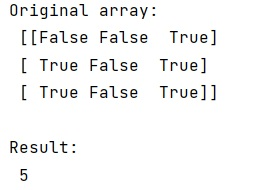# How to count the number of true elements in a NumPy bool array?

Learn how to count the number of true elements in a NumPy bool array?
Submitted by Pranit Sharma, on December 21, 2022

NumPy is an abbreviated form of Numerical Python. It is used for different types of scientific operations in python. Numpy is a vast library in python which is used for almost every kind of scientific or mathematical operation. It is itself an array which is a collection of various methods and functions for processing the arrays.

## Counting the number of true elements in a NumPy bool array

In programming, we sometimes use some specific values that only have two values, either True or False. These values are known as Boolean values.

A NumPy array containing bool type values is considered as a bool array, suppose that we are given a bool array and we need to count how many true values are present in this array.

For this purpose, we will directly use the sum method, here the important point to understand is that a bool array contains true or false values true has a value of 1 and false has a value of 0 and if we add al the true values and false values together, we will get an integer value resulting the total number of true values.

Let us understand with the help of an example,

## Python code to count the number of true elements in a NumPy bool array

```# Import numpy
import numpy as np

# Creating a numpy array
arr = np.array([
[0, 0, 1],
[1, 0, 1],
[1, 0, 1]],
dtype=np.bool)

# Display original array
print("Original array:\n",arr,"\n")

# Getting the count of true values
res = arr.sum()

# Display Result
print("Result:\n",res)
```

Output: